Skip to content

# What is Powder Flow Analysis?

"Learn About Powder Flow" is a white paper published by Brookfield that describes powder flow behavior and the resulting problems when powder flow is impeded.

LEARN ABOUT POWDER FLOW

Problems with Powder Relating to Gravity Flow Behavior
A typical industrial powder processing line will include several storage vessels (e.g. bins, bunkers, silos, hoppers, Intermediate Bulk Containers or IBCs, sacks etc), feeding or handling steps (e.g. belt conveyor, screw conveyor, pneumatic conveyor, gravity chutes etc) and processing steps (e.g. milling, mixing, drying, bagging etc). A major industrial problem is getting the powder to discharge reliably from storage into the next process step. Therefore, to understand the application of powder flow measurements, it is useful to have some background knowledge of the flow patterns and flow obstructions that can occur inside the storage vessels on a processing line.

What are the Powder Flow Patterns that can Occur in a Process Storage Vessel?
Principally there are two different flow patterns that can occur:

Core-flow (shown in Figure 1a) can be considered the default flow pattern and is characterized by powder discharge through a preferential flow channel above the draw down point of the outlet. Powder is drawn into the flow channel from the top free surface of the inventory. This gives a first-in last-out discharge regime and, if operated on a continuous (rather than batch) mode, the powder around the walls in the lower section will remain static in the vessel until the time that it is drained down to empty.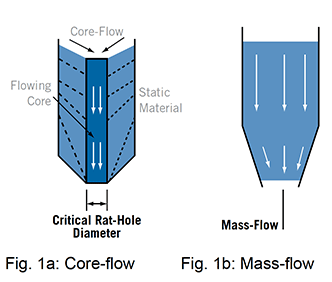Mass-flow (shown in Figure 1b) is the desirable flow pattern for powders that are poor flowing or time sensitive, but must be specifically designed for. Here the entire contents of the vessel are ‘live’, giving a first-in first-out discharge regime. To achieve this, the hopper walls must be sufficiently steep and smooth.  For a given wall material/converging angle, the powder wall friction must be below a critical value.  Also, the product discharge must be controlled by a valve or feeder that allows powder to flow through the entire cross sectional area of the outlet.  (It is this final point that prevents many vessels from operating in mass-flow.)

A wall friction test will be able to give an approximate assessment of whether a given hopper geometry will support mass-flow (with the proviso that the outlet area is fully active).  For an exact calculation of the maximum mass-flow hopper half angle, both wall friction and flow function tests must be undertaken.

What are the Powder Obstructions that Can Occur to Prevent Flow:
Principally, there are two flow obstructions that can occur: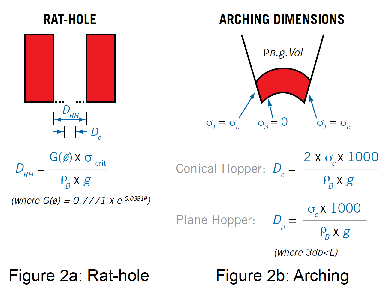‘Rat-holing’ (shown in Figure 2a) is the principle flow obstruction in a core-flow vessel where the powder in the flow-channel above the outlet discharges and leaves a stable internal structure.

Arching (shown in Figure 2b) is the flow obstruction in a mass-flow vessel, where a stable powder arch forms across the outlet or converging walls of the hopper, thereby preventing flow.

For a given powder there is a critical outlet dimension that must be exceeded to ensure reliable discharge of a core-flow or mass-flow vessel.  These are the critical rat-hole diameter Drh and the critical arching diameter DC or Dp (depending on the hopper geometry – see Figure 3).  The AMETEK Brookfield Powder Flow Tester (PFT) can calculate these critical dimensions following a flow function measurement.  An accurate dimension requires a wall friction test as well.  Note that for a given powder the rat-hole diameter is significantly larger than the arching diameter.

Key Differences Between Powders and Fluids

For Newtonian fluids the resistance to shear (viscosity) is independent of the normal pressure but dependent on the shear rate.  In powders the effect of these factors is reversed so that shear stress of a powder is strongly dependent on the normal stress but independent of the shear rate.  Hence, when characterizing powders, test are undertaken at a single speed but over a range of normal stresses.  The other key difference is that powders are anisotropic so the stresses are not equal in all directions and are frictional so that they can generate shear stresses at wall boundaries (see wall friction section).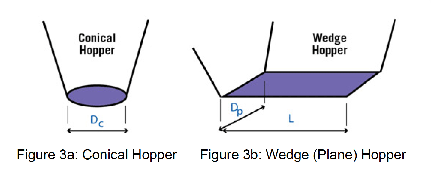Flow Function Test
The primary measure of powder flowability is the powder flow function - which gives a measure of the amount of strength the material retains at a stress free surface following consolidation to a given stress level.  The simplest way of explaining the flow function is with the uniaxial unconfined failure test shown in Figure 4, which measures the strength of a free standing column of powder.  This condition is analogous to the condition of the powder arch across a hopper outlet shown in Figure 2b.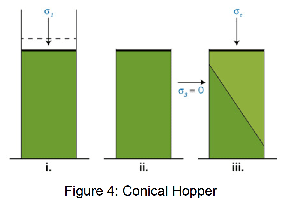1) Consolidation  of sample.
Powder is placed in a cylindrical cell and compacted under a normal stress s1.
2) Unconfined sample.
The mould is now carefully removed to reveal a compacted column of powder.
3) Unconfined failure of sample.
The normal stress acting on the column of powder is gradually increased until failure occurs, and the peak normal stress sc is recorded.

The uniaxial unconfined failure test is conducted over a range of consolidation stresses and the flow function is con-structed by plotting the unconfined failure strength versus the consolidation stress as shown in Figure 5.  The greater the flow factor (ff) value, the more free-flowing the powder (Table 1).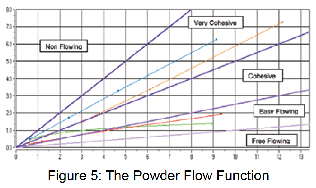Table 1: Standard Classification of Powder Flowability

Anticipated Uses of the Brookfield Powder Flow Tester

Bench marking - Measure flow properties on all raw powders and blends to determine if there are differences in their flowability and whether these correspond with plant experience.

New materials
- Test new ingredients/blends versus existing ingredients /blends to determine whether the alternative material is likely to be easier or more difficult to handle.  This potential material handling cost can be factored into the purchasing decision.

Reverse engineering - If you have plant experience with powders on a given process line, you can use the PFT to determine the flow properties of each powder and use this as a flowability reference for future batches.

Design - Design the geometry (converging angle and outlet size) of new hoppers/silos for reliable flow.

Alternative Methods of Displaying the Flow Function Test Results

To demonstrate powder flowability, the flow function can be presented graphically (as in Figure 5) to describe behaviour over the stress range of approximately 0.3kPa to 13kPa.  This stress range is representative of that experienced by the powder in small to intermediate sized silos.  However, describing flowability with a function may complicate the analysis as it is sometimes found that the flow functions of two different materials cross over one another, so that their relative ranking changes with stress levels.  Alternatively, flowability rankings for specific stress levels can be determined by calculating the following parameters:

Estimated Critical Arching diameter [m]: The minimum silo outlet size for reliable gravity discharge in mass-flow, calculated using the arching equation in Figure 2b.  The stress value is the intercept of the flow function with an ff =1.4 line.  This is the default flow factor settings but can be adjusted by the user within a 1.0 to 1.8 range for silo design applications.

Estimated Critical ‘Rat-hole’ diameter in [m]: The minimum outlet diameter to prevent the formation of a stable ‘rat-hole’ in a core-flow vessel.  The outlet diameter is calculated using the rat-holing equation in Fig 2a.  The stress value is the intercept of the flow function with an ff=2.5 line and can be user-set to any stress level.

Flow index
: The gradient of a line from the origin to the last point on the flow function, typically in the range of 0.1 to 1.0.  This index will give a comparison of materials behaviour at intermediate compaction stresses greater than one meter depth of powder.

Flow intercep
t: The intercept of the best fit linear failure function with the unconfined failure strength axis giving a number in kPa.  This gives a number that reflects powder flowability at compaction stresses typically less than 0.15m depth of powder.

Note that a time consolidated flow function test allows the user to investigate whether the material gains strength during long term storage.

Wall Friction Test

The friction acting at the wall/powder interface has a significant influence on the stress distribution within processing vessels, silos and hoppers.

The higher the wall friction, the more the powder weight is transferred down through the silo/vessel/container walls, rather than compacting the bulk solid below. The lower the friction, the more the powder self-weight is transmitted through the bulk solid. This ‘Janssen effect’ is illustrated in Figure 6, which demonstrates how the vertical pressures in the vertical section of a silo would vary if the wall friction were increased from zero to a large value of 400. The presence of the wall friction has a negative feed-back effect on the pressure increase with depth, so generally the stresses approach constant values at a depth of approximately 4 vessel diameters.Software can be used to estimate pressures in a container based on measurements of the bulk density ρ, wall friction, internal friction δj and container diameter D. The principal consolidation pressure σ1 at depth Z is given by the equation:

The wall friction anglerepresents the angle to which a wall surface must be inclined as shown in Figure 7 to cause powder to slip.  The wall friction angle is typically in the range of 10 to 45 degrees. The wall friction angle is also called the chute angle.The wall friction angle is also called the chute angle.

While the results of the wall friction test can be displayed graphically in the form of a wall failure locus as shown in Figure 8a (representing the limiting shear stress the powder can support at a wall), or the form of a wall friction angle function as shown in Figure 8b (representing how the wall friction angle changes with reducing stress), one of the following four flow indices derived from the maximum wall friction locus are usually adequate.  These wall failure properties are:θcθp = The maximum mass-flow hopper half angle (measured to the vertical) for conical or planar hoppers.= The maximum wall friction angle to determine the minimum chute angle for gravity flow (see Figure 8b).

Grad  = The maximum wall friction angle displayed as a coefficient.

cw = The wall cohesion shear stress in kPa that can be supported at the wall under zero normal stress (see Figure 8a). This determines the ‘stickiness’, i.e. whether powder is likely to stick to the wall surface under close to zero stress. i.e. will powder build up on the walls of the chutes around discharge/transfer points.

An extended wall friction test allows the wall sample to be subject to large shear displacements (on the order of 30 meters) to establish whether long term powder build up on the wall would be expected.

Bulk Density Test

It is the self-weight of the powder, its bulk density, that controls the stresses acting on the powder when flowing or when static in processing lines/ silos etc.  The bulk density is measured during the course of the flow function test (and is required to calculate the critical outlet dimensions) and the wall friction test, but it can also be measured in a separate single test for bulk density alone.

The bulk density is commonly displayed as a bulk density curve (Figure 9).  Generally a free flowing material will be incompressible- so will show only a small increase in density with stress.  A very cohesive, poorly flowing bulk solid by comparison will show a large increase in bulk density with increasing stress.ρfill = The fill bulk density to be expected when the powder is poured into a container

ρcomp = The compacted bulk density will give an indication of the bulk density to expect if the material is poured and compacted to high stress

Summary

The Brookfield Powder Flow Tester offers four standard tests:

Flow Function Test - Measures internal strength, flow function, internal friction function and bulk density function- used for characterizing the flow strength and arching/ rat-holing potential of powders.

Time Consolidated Flow Function Test - Same as above but following static storage for a user defined time period.

Wall Friction Test - Measures friction between the powder and a given wall surface and the bulk density function - used for assessing mass-flow hopper half angles and gravity flow chute angles.

Bulk Density Test - Measures bulk density curve of the powder.

Note that to undertake a full silo design requires the user to run and combine the results of tests 1, 2 and 3.